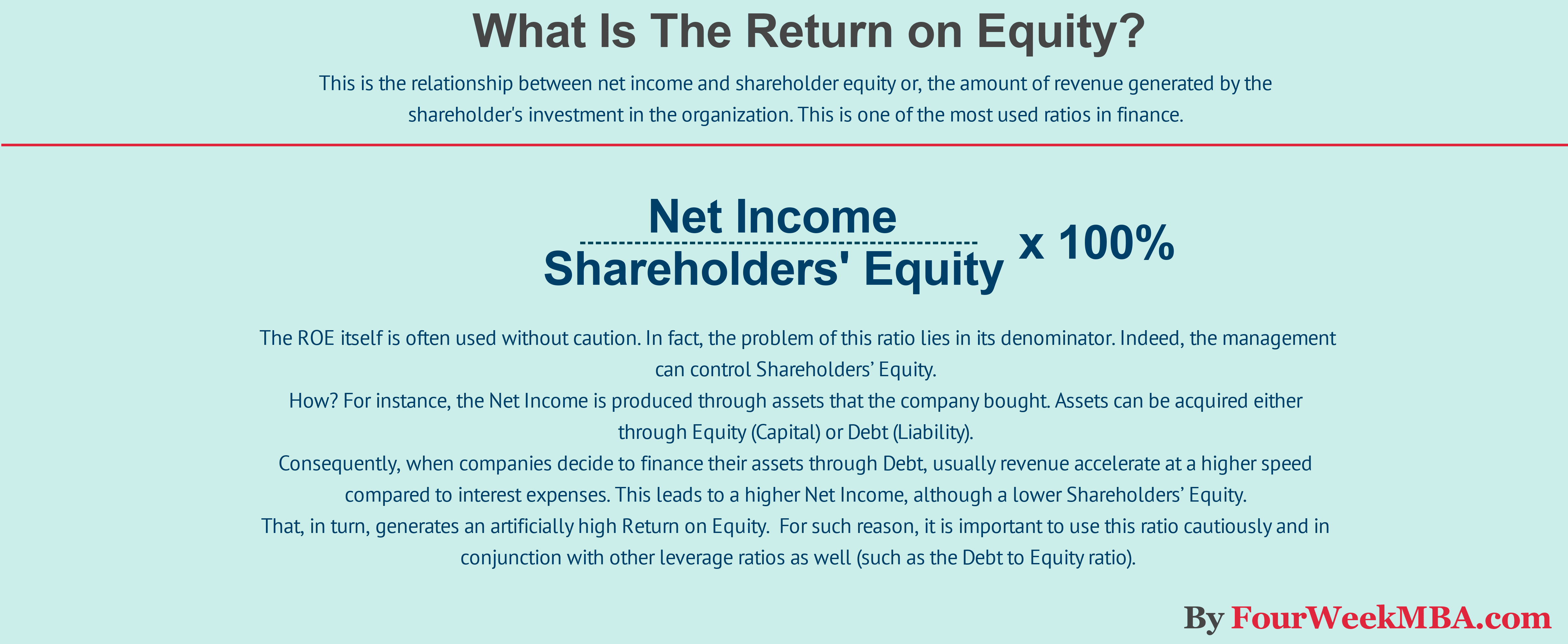# 15 Financial Ratios Formulas To Analyse Any Business

These are the most important financial ratios formulas you can use to analyze any business:

## What is a current ratio?## What is a quick ratio?## What is the absolute ratio?## What is the accounts receivable turnover ratio?## What is the accounts payable turnover ratio?## What is the inventory turnover ratio?## What is a debt to assets ratio?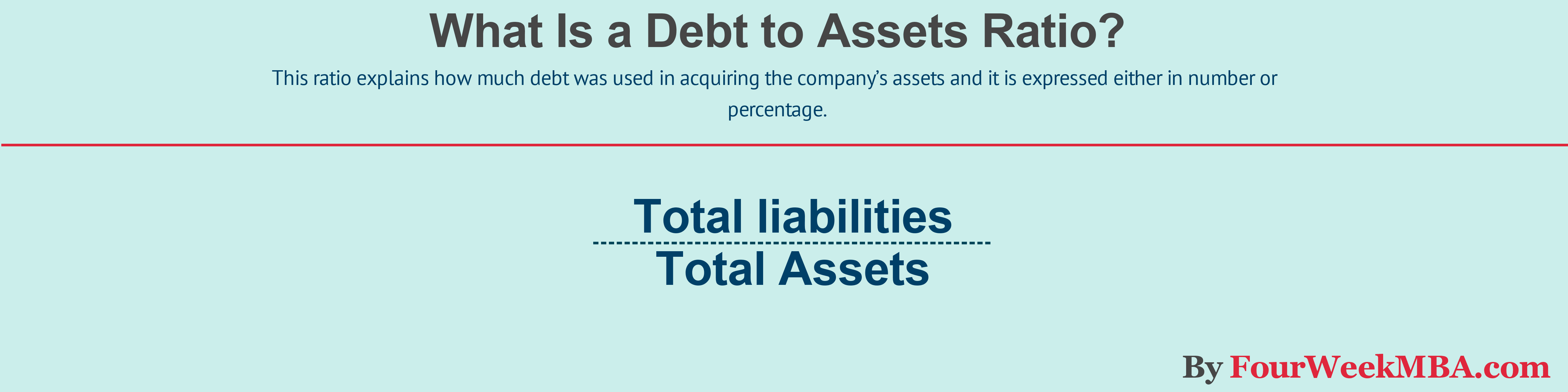## What is a debt to equity ratio?## What is the interest coverage ratio?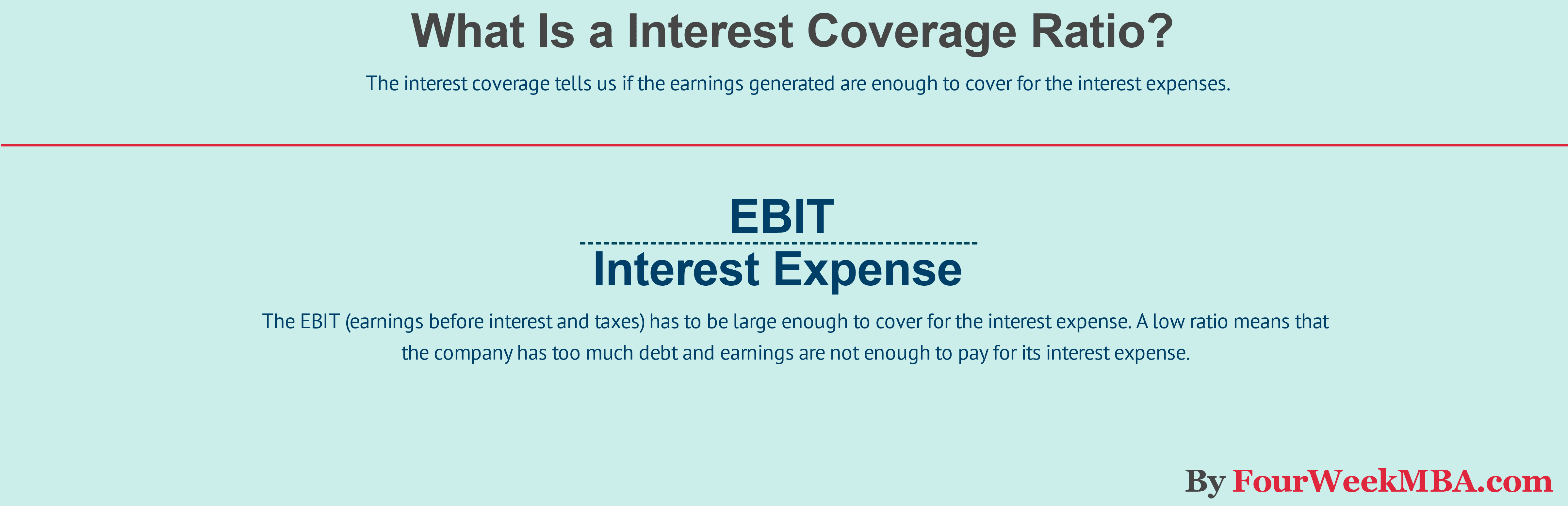## What is a gross profit margin?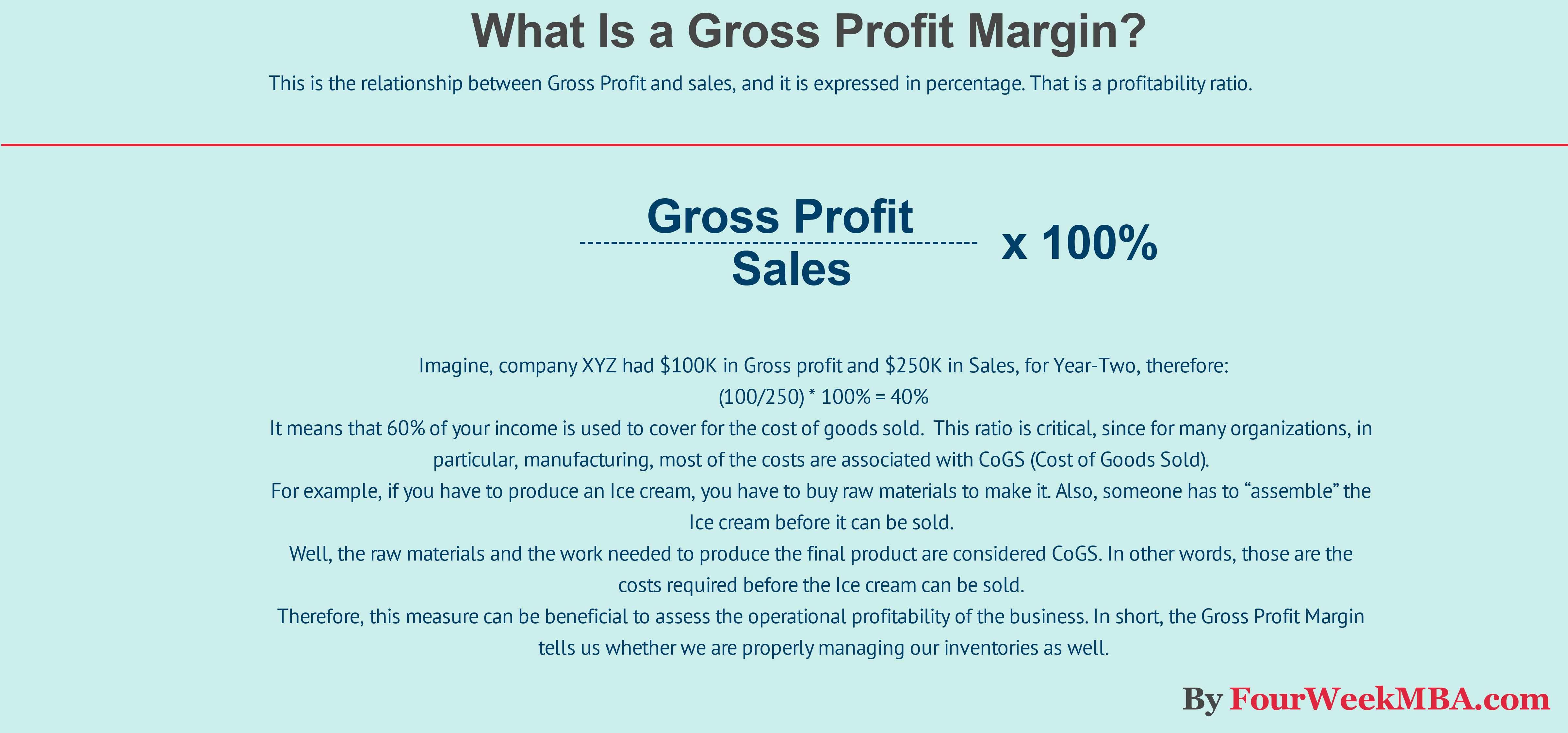## What is an operating profit margin?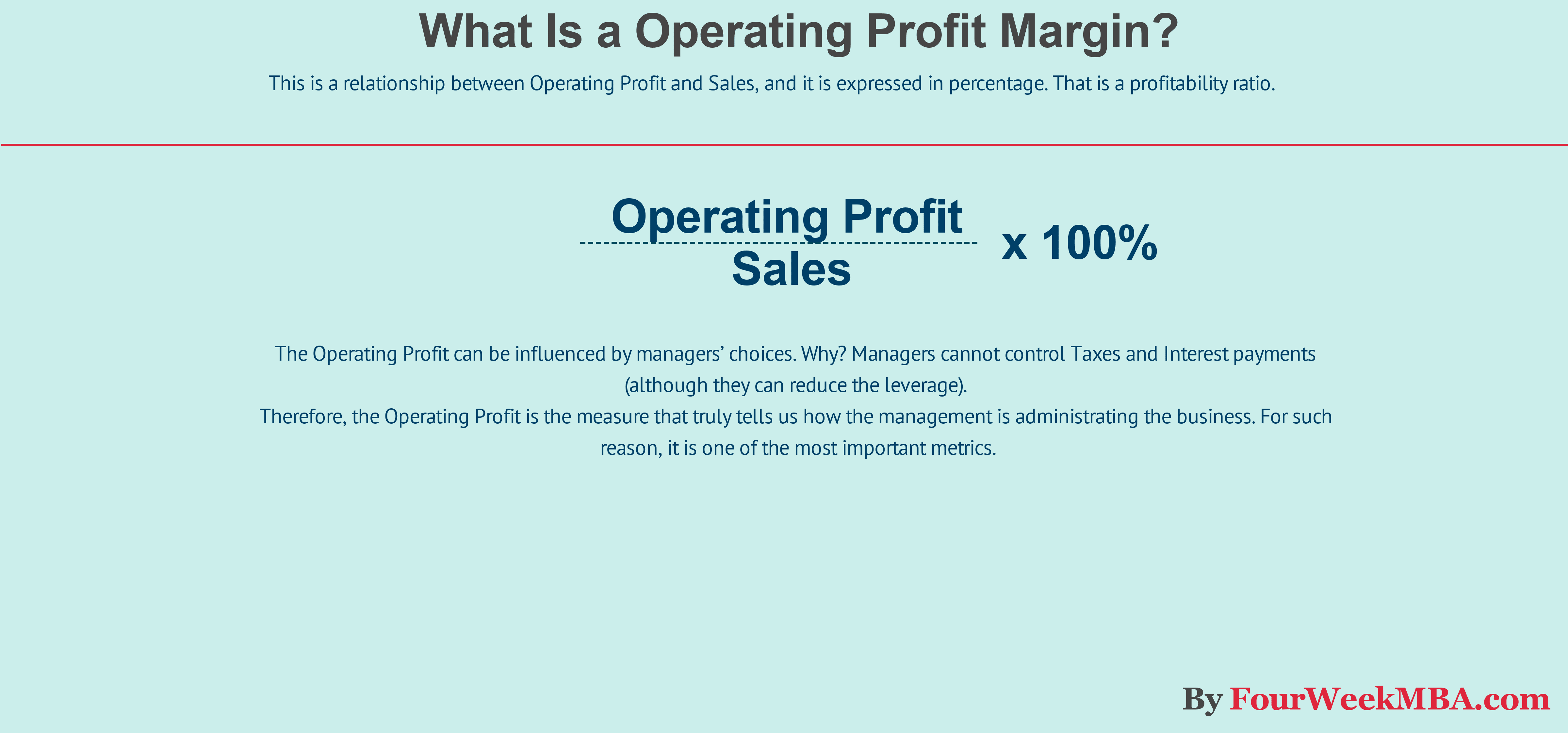## What is a return on capital employed?## What is the return on equity?## What is the earning per share ratio formula?

This is given by:

(Net Income – Preferred Dividends) / Weighted Average Number of Common Shares

## What is the price/earnings ratio formula?

This is given by:

(Net Income – Preferred Dividends) / Weighted Average Number of Common Shares